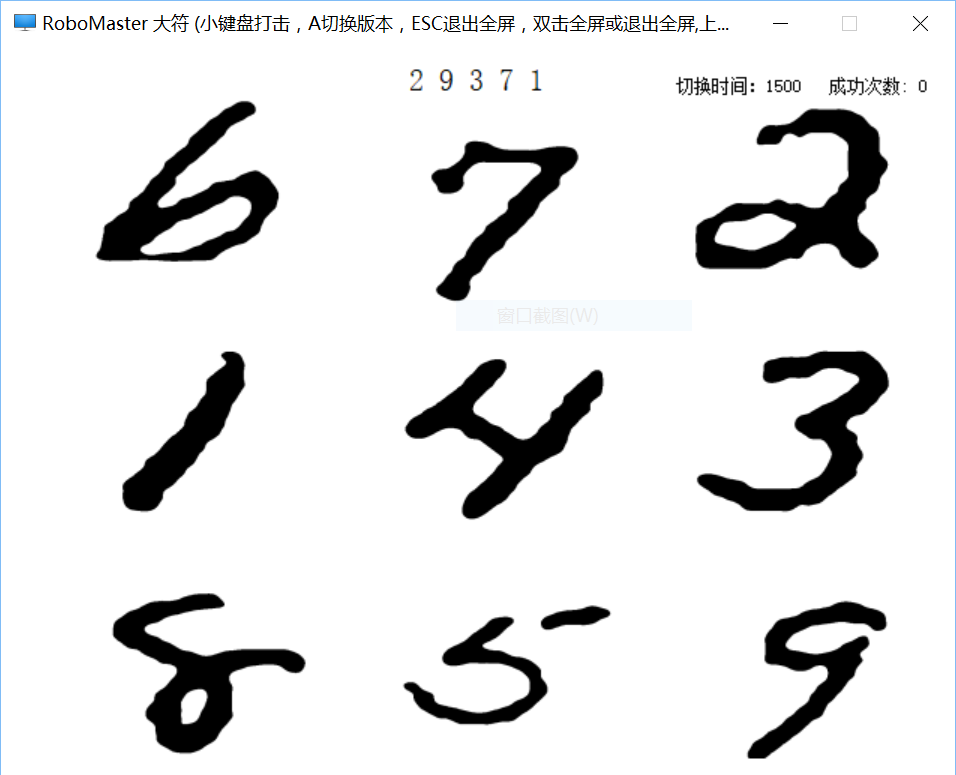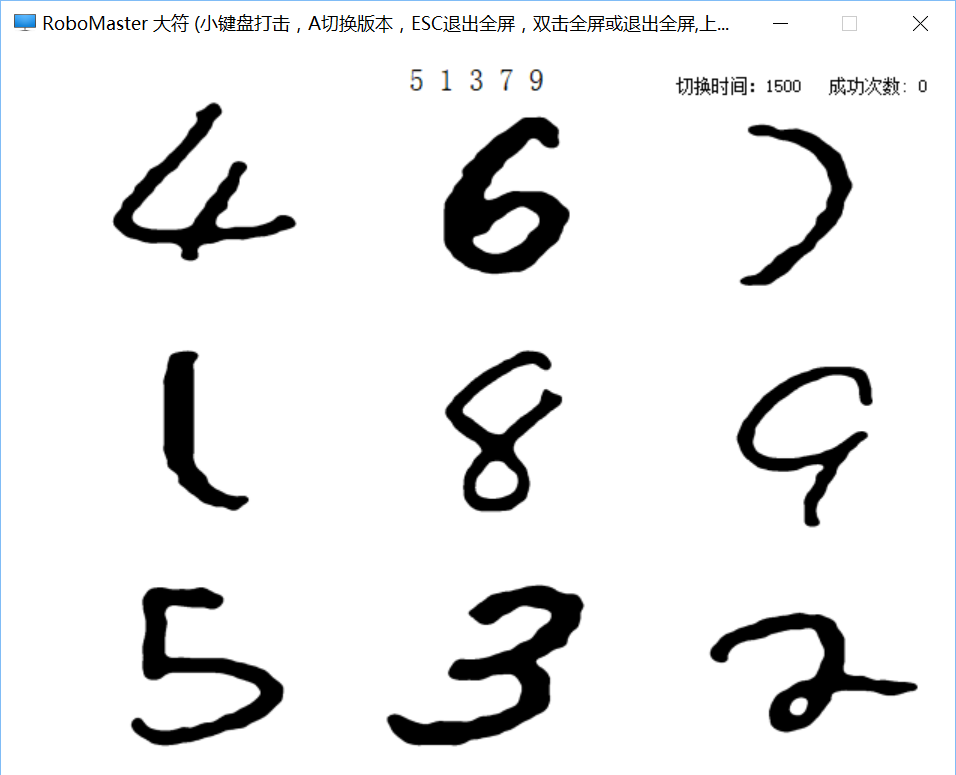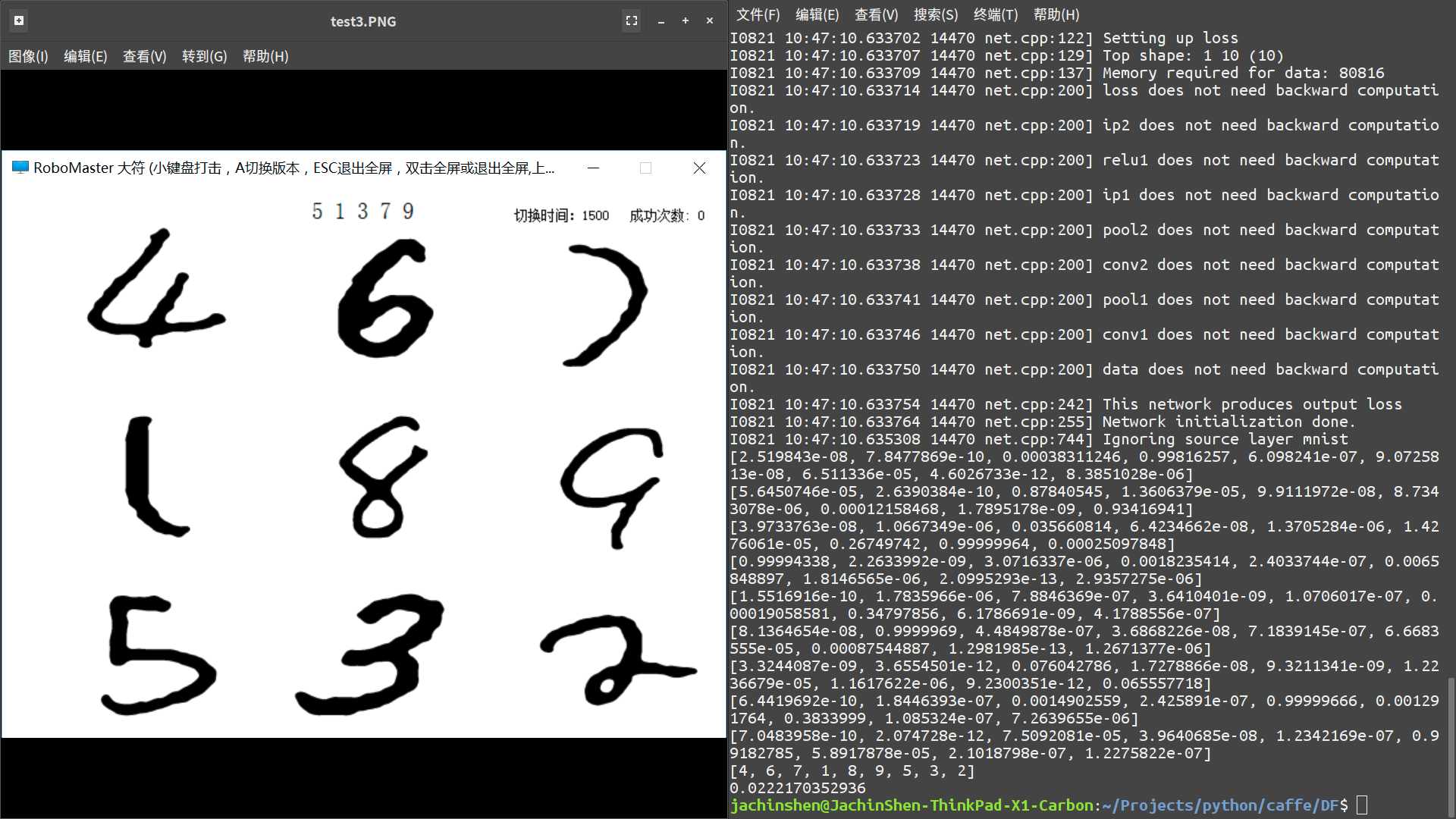Jachin Shen

# 部署 Caffe 深度学习模型

Caffe 是一个开源的深度学习框架，它通过文本文件构建卷积神经网络的结构，可以在 Python 和 C++ 中部署。

## 输入层和输出层结构修改

layer {
name: "mnist"
type: "Data"
top: "data"
top: "label"
include {
phase: TEST
}
transform_param {
scale: 0.00390625
}
data_param {
source: "examples/mnist/mnist_test_lmdb"
batch_size: 100
backend: LMDB
}
}


layer {
name: "data"
type: "Input"
top: "data"
input_param{ shape: { dim: 1 dim: 1 dim: 28 dim: 28 } }
}


layer {
name: "loss"
type: "SoftmaxWithLoss"
bottom: "ip2"
bottom: "label"
top: "loss"
}


layer {
name: "loss"
type: "Softmax"
bottom: "ip2"
top: "loss"
}


## Python

### 加载网络

model = './lenet_deploy.prototxt'#读取模型
weights = './lenet_iter_10000.caffemodel'#读取参数
caffe.set_mode_cpu;#设置成cpu模式，可改成gpu
net = caffe.Net(model, weights, caffe.TEST)#加载网络


Net 构造中的最后一个参数表示网络属于 TEST ，即测试阶段，这有可能影响网络的结构，需要确保在网络结构文本文件中，测试阶段的模型适合部署，特别是输入层和输出层。

### 图像预处理img_raw = cv2.imread('test1.PNG',0)#读灰度图
_,img_raw = cv2.threshold(img_raw, 128, 255, cv2.THRESH_BINARY_INV)#二值化
img_raw = cv2.normalize(img_raw.astype('float'), None, 0.0, 1.0, cv2.NORM_MINMAX)
#归一化


### 提取数字区域并前传预测

results = [[0 for col in range(10)] for row in range(9)]#中间结果矩阵
result = [0,0,0,0,0,0,0,0,0]#最终结果矩阵
for i in range(0,3):
for j in range(0,3):
img = img_raw[100+225*i:325+225*i,318*j:318+318*j]#截取测试图中九宫格部分
img = cv2.resize(img, dsize=(28, 28), interpolation = cv2.INTER_LINEAR )
#压缩为28*28大小
img = img.reshape((1,1,28,28))#转换为1*1*28*28维向量，便于数据输入
net.blobs['data'].data[...] = img     #将图片载入到blob中
out = net.forward()#前传
results[3*i+j] = net.blobs['loss'].data.flatten()
#取出最后一层（Softmax）属于某个类别的概率值
for i in range(1,10):
tmp = [0,0,0,0,0,0,0,0,0]
for j in range(0,9):
tmp[j] = results[j][i]
print(tmp)
result[tmp.index(max(tmp))] = i#取出每个数字最大概率所在的九宫格位置
print(result)## C++

Caffe 本来就是用 C++ 写成的，所以只要调用它的 C++ 函数就可以实现部署了。

### 两个函数

int get_blob_index(boost::shared_ptr< Net<float> > & net, char *query_blob_name)
{
std::string str_query(query_blob_name);
vector< string > const & blob_names = net->blob_names();
for( unsigned int i = 0; i != blob_names.size(); ++i )
{
if( str_query == blob_names[i] )
{
return i;
}
}
return -1;
}


void caffe_forward(boost::shared_ptr< Net<float> > & net, float *data_ptr)
{
//get the id of data layer from the name"data"
//you can replace the name with your own data layer or any layer you want to put data
char query_blob_name = "data"; /* data, conv1, pool1, norm1, fc6, prob, etc */
unsigned int blob_id = get_blob_index(net, query_blob_name);

//or, you can directly give the blob_id, like blob_id=0;(0 is common for data layer)

cout<<"data blob id:"<<blob_id<<endl;
Blob<float>* input_blobs = net->input_blobs()[blob_id];
switch (Caffe::mode())
{
case Caffe::CPU:
memcpy(input_blobs->mutable_cpu_data(), data_ptr,
sizeof(float) * input_blobs->count());
break;
//if you haven't installed CUDA, comment this case
case Caffe::GPU:
cudaMemcpy(input_blobs->mutable_gpu_data(), data_ptr,
sizeof(float) * input_blobs->count(), cudaMemcpyHostToDevice);
break;
//comment to here
default: break;
}
//net->ForwardPrefilled();
net->Forward();
}


### 主函数

#### 加载模型

char proto = "your_path/lenet_deploy.prototxt"; /* 加载CaffeNet的配置 */
Phase phase = TEST; /* or TRAIN */
Caffe::set_mode(Caffe::CPU);
boost::shared_ptr< Net<float> > net(new caffe::Net<float>(proto, phase));
char model = "your_path/lenet_iter_10000.caffemodel";//load arguments of model
net->CopyTrainedLayersFrom(model);


#### 处理图片resize(img, img, Size(28, 28));
threshold(img, img, 128, 1, THRESH_BINARY_INV);



1. 输入层要求 32 位浮点类型的数据，但是图片是以 8 位整数储存的；

2. 数据的传入是通过指针的，所以要求数据连续存放，但是 Mat 的数据有可能是分散的。


float data_ptr;//for data
uchar* p_data;
for( int i=0; i<28; i++)
{
p_data = img.ptr< uchar>(i);
for(int j=0; j<28; j++)
{
data_ptr[28*i+j]=(float)*(p_data+j);//get data and convert to float
}
}



#### 前传并取出预测值

caffe_forward(net, data_ptr);

char query_blob_name = "loss"; /* data, conv1, pool1, norm1, fc6, prob, etc */
unsigned int blob_id = get_blob_index(net, query_blob_name);

boost::shared_ptr<Blob<float> > blob = net->blobs()[blob_id];
unsigned int num_data = blob->count();
std::cout<<"data number:"<<num_data<<std::endl;
const float *blob_ptr = (const float *) blob->cpu_data();//get pointer to result

for( int i=0; i<num_data; i++)
{
cout<<*(blob_ptr+i)<<" ";
}


# 总结

1. 整个流程为：加载网络 -> 处理图片 -> 数据格式转化 -> 传入数据 -> 前传 -> 取出预测的概率。

2. 主要问题在于数据格式的转化，要从图片的数据转化为 Caffe 可以处理的数据。python 中因为数据类型简单，所以比较方便；c++ 中数据类型很严格，要进行强制类型转化。